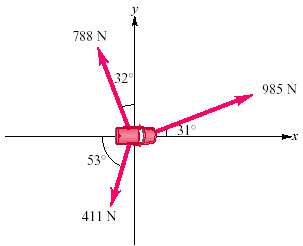Physics Practice Problems Vector Addition & Subtraction Practice Problems Solution: Workmen are trying to free an SUV stuck in the mud...

⚠️Our tutors found the solution shown to be helpful for the problem you're searching for. We don't have the exact solution yet.

# Solution: Workmen are trying to free an SUV stuck in the mud. To extricate the vehicle, they use three horizontal ropes, producing the force vectors shown in the figure.Find the y components of each of the three pulls.Use the components to find the magnitude of the resultant of the three pulls.Find the x components of each of the three pulls.Use the components to find the direction of the resultant of the three pulls.

###### Problem

Workmen are trying to free an SUV stuck in the mud. To extricate the vehicle, they use three horizontal ropes, producing the force vectors shown in the figure.Find the y components of each of the three pulls.

Use the components to find the magnitude of the resultant of the three pulls.

Find the x components of each of the three pulls.

Use the components to find the direction of the resultant of the three pulls.

#### Q. Two forces have the same magnitude F.What is the angle between the two vectors if their sum has a magnitude of 2F?What is the angle between the two ve...

Solved • Mon Oct 29 2018 18:50:27 GMT-0400 (EDT)

#### Q. Vector A⃗ vec{A} has components Ax = 1.45 cm, Ay = 2.20 cm; vector B⃗ vec{B}has components Bx= 4.00 cm, By= -3.60 cm.Find the x-component of the vecto...

Solved • Mon Oct 29 2018 18:50:16 GMT-0400 (EDT)